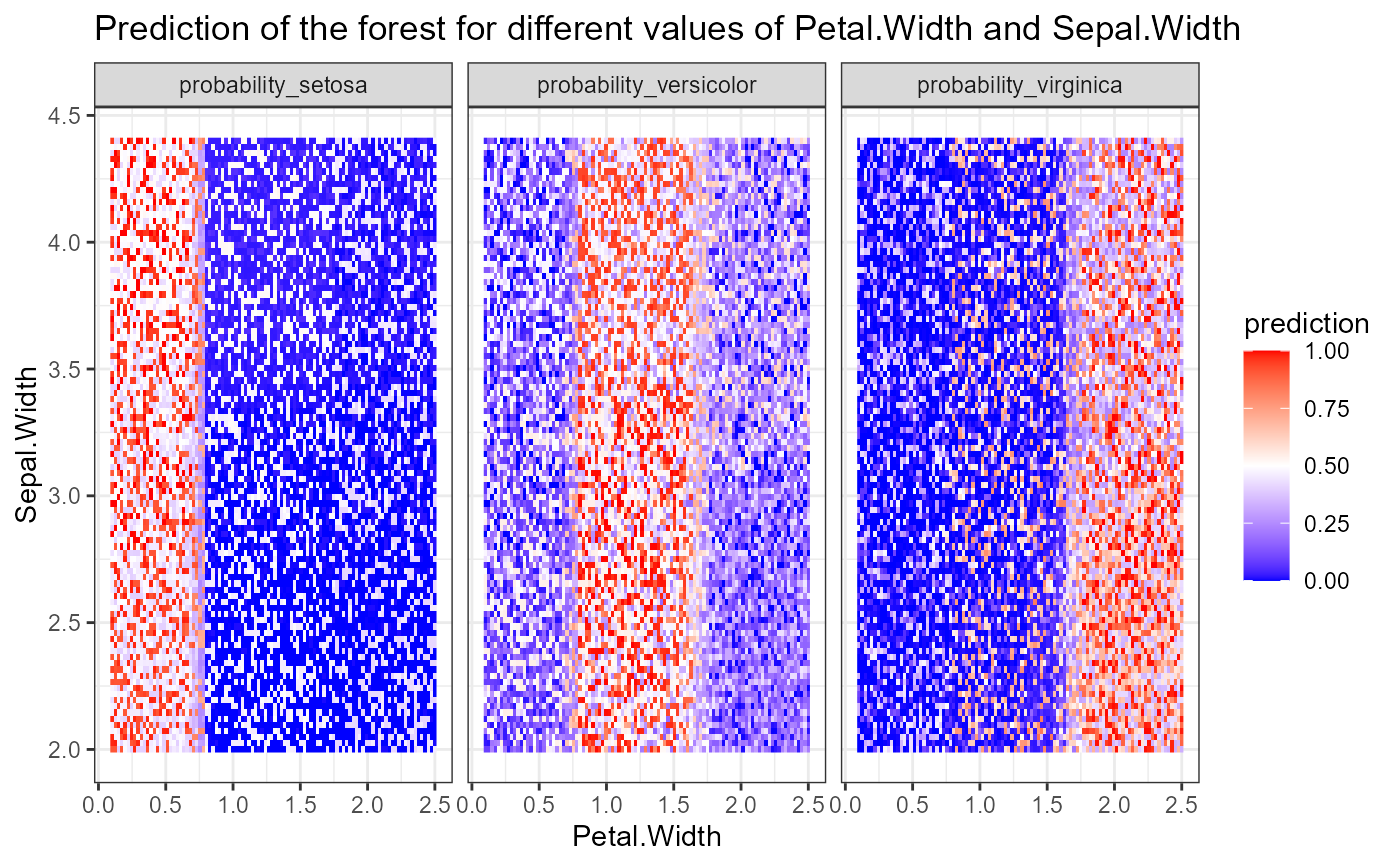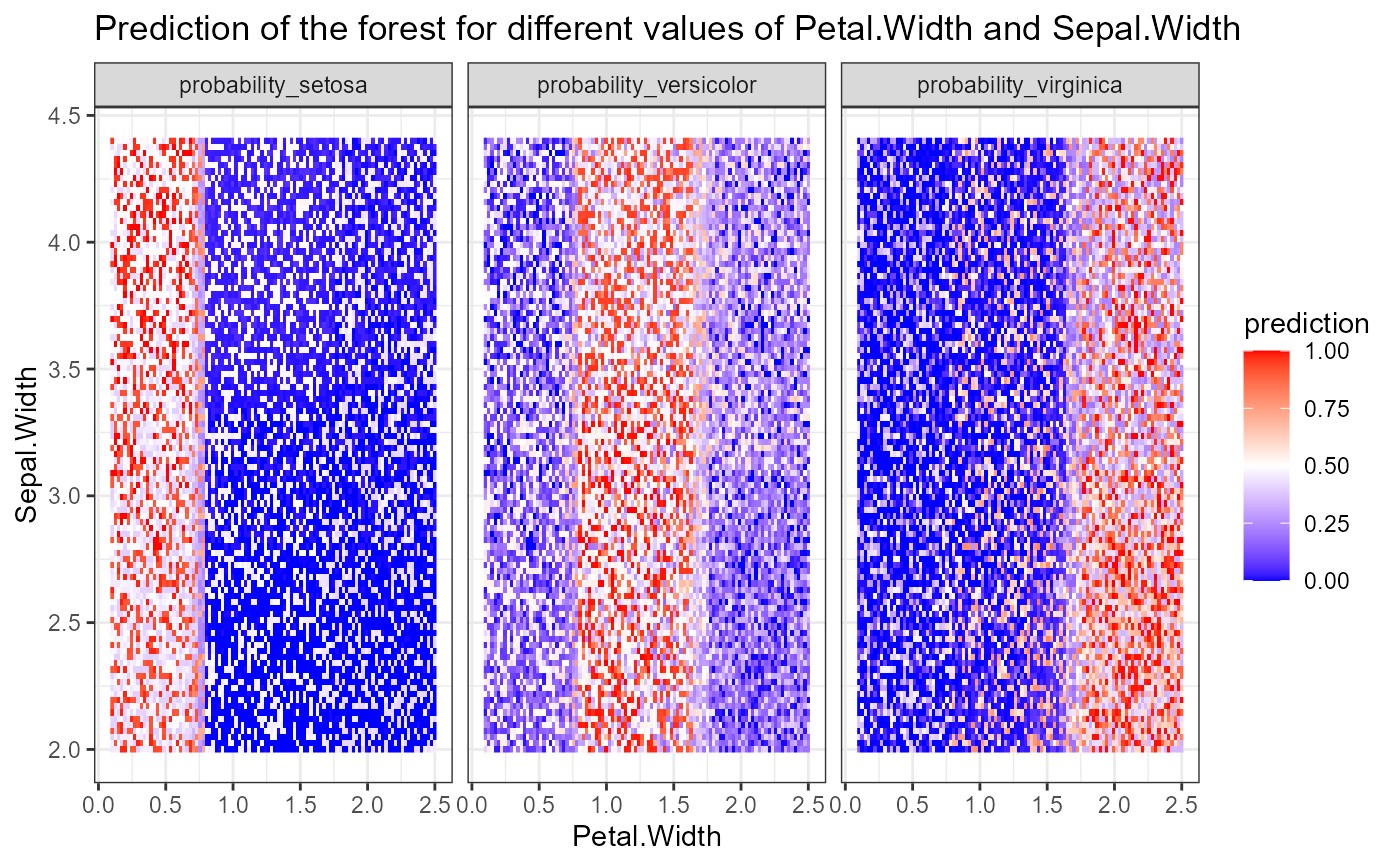Plot the prediction of the forest for a grid of values of two numerical variables

plot_predict_interaction(
forest,
data,
variable1,
variable2,
grid = 100,
main = paste0("Prediction of the forest for different values of ", paste0(variable1,
paste0(" and ", variable2))),
time = NULL
)

## Arguments

forest A randomForest or ranger object The data frame on which forest was trained A character string with the name a numerical predictor that will on X-axis A character string with the name a numerical predictor that will on Y-axis The number of points on the one-dimensional grid on x and y-axis A string to be used as title of the plot A numeric value specifying the time at which to predict survival probability, only applies to survival forests. If not specified, the time closest to predicted median survival time is used

A ggplot2 object

## Examples

forest <- randomForest::randomForest(Species ~., data = iris)
plot_predict_interaction(forest, iris, "Petal.Width", "Sepal.Width")forest_ranger <- ranger::ranger(Species ~., data = iris)
plot_predict_interaction(forest, iris, "Petal.Width", "Sepal.Width")